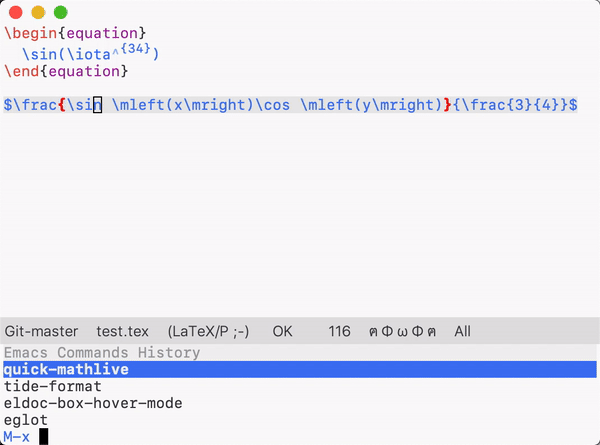# Emacs所见即所得地编辑数学公式（伪）

#1#2

#3

#4

#5

#6

github上有人想让我把Guppy 整合进emacs-webkit-katex-render 暂时还没空做。。。 有个问题是linux上的xwidget文字输入体验过于糟糕。。。mac上倒是挺好

#7

mac下的xwidget可以这样获得：

brew edit emacs-plus

https://gist.github.com/fuxialexander/67ce2d87543a8ec8f76a4868ea93e5b7

#8

#9

#10

#11

#12

#13

AucTeX有LaTeX-math-mode可以用（貌似叫这个），就是用~键快速输入数学符号，因为我大量用latex，所以常用的符号都自定义在这里了，输入很快，差不多能满足快速作笔记的要求了。

#14

\documentclass[class=scrreprt]{standalone}
\usepackage{varwidth}

\usepackage[utf8]{inputenc}
\usepackage[T1]{fontenc}
% \KOMAoptions{fontsize=24pt}
% \usepackage{times,newtxmath}
\usepackage{lmodern}

\usepackage{latexsym, amsmath, amssymb, amsfonts, mathrsfs, arydshln, mathtools}
\usepackage{algorithm, algpseudocode}
\usepackage{graphics, setspace}

\begin{document}
\begin{varwidth}{10in}
\begin{equation*}
\begin{aligned}
% insert equation here:
% Equation #1

\end{aligned}
\end{equation*}
\end{varwidth}
\end{document}


#15

#16

focus到emacs这个，之前在坛里讨论过，记得好像是从magit抄的：

(defun open-emacs-window ()
"Switch to emacs frame."
(select-frame-set-input-focus (selected-frame)))

mg() {
emacsclient -n -e '(magit-status)' -e '(open-emacs-window)' > /dev/null
}
`